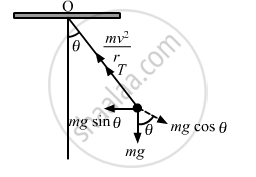Share

# Suppose the Bob of the Previous Problem Has a Speed of 1.4 M/S When the String Makes an Angle of 0.20 Radian with the Vertical. Find the Tension at this Instant. - Physics

ConceptCircular Motion

#### Question

Suppose the bob of the previous problem has a speed of 1.4 m/s when the string makes an angle of 0.20 radian with the vertical. Find the tension at this instant. You can use cos θ ≈ 1 − θ2/2 and SINθ ≈ θ for small θ.

#### Solution

Given:
Mass of the bob = m = 0.1 kg
Length of the circle = R = 1 m
Velocity of the bob = v = 1.4 m/s
Let T be the tension in the string when it makes an angle of 0.20 radian with the vertical.From the free body diagram, we get :

$T - \text{mg}\cos\theta = \frac{\text{mv}^2}{\text{R}}$

$\text{T} = \frac{\text{mv}^2}{\text{R}} + \text{mg} \cos\theta$

$\text { For small } \theta, \text {it is given that : }$

$\cos\theta = 1 - \frac{\theta^2}{2}$

$\therefore T = \frac{0 . 1 \times (1 . 4 )^2}{1} + (0 . 1) \times 9 . 8\left( 1 - \frac{\theta^2}{2} \right)$

$= 0 . 196 + 0 . 98 \times \left( 1 - \frac{\left( 0 . 2 \right)^2}{2} \right)$

$= 0 . 196 + 0 . 9604$

$= 1 . 156 N \approx 1 . 16 \text{ N }$

Is there an error in this question or solution?

#### APPEARS IN

Solution Suppose the Bob of the Previous Problem Has a Speed of 1.4 M/S When the String Makes an Angle of 0.20 Radian with the Vertical. Find the Tension at this Instant. Concept: Circular Motion.
S